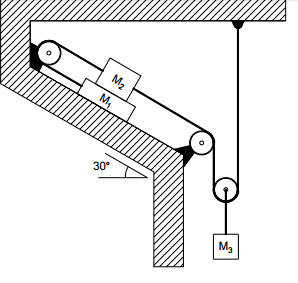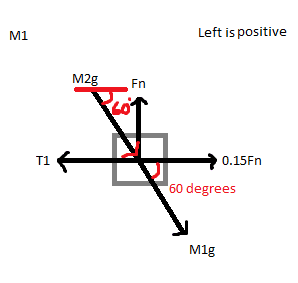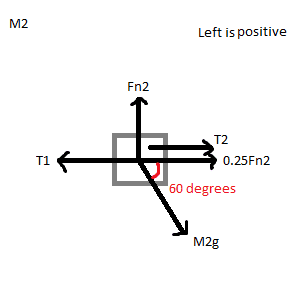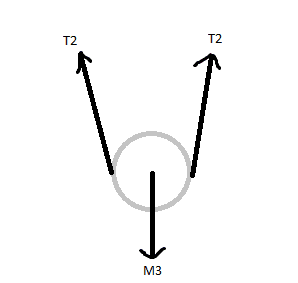# Static Equilibrium Pulley Problem

• Sixth42

## Homework StatementThe attachment above contains the diagram given in the problem

M1 = 25kg
M2 = 35kg
Friction coefficient between M1 and stucture = 0.15
Friction coefficient between M1 and M2 = 0.25
Friction on pulleys and mass on pulleys is negligible.

Find the minimum mass M3 for sliding to occur in the system (i.e. the maximum mass for which the system will remain in static equilibrium).

∑Fx = 0
ΣFy = 0

## The Attempt at a Solution

For the first mass:The above attachment contains the diagram I used with the rotated coordinate system. My equations were:
∑Fy = 0
-M2g sin(60°)-M1g sin(60°) + Fn = 0
Fn = 509.7N

∑Fx = 0
-M2g cos(60°)-M1g cos(60°) - 0.15Fn + T1 = 0
T1 = 370.8N

For the second mass:The above attachment contains the diagram I used with the rotated coordinate system. My equations were:
∑Fy = 0
-M2g sin(60°) + Fn2 = 0
Fn2 = 297.3N

∑Fx = 0
-M2g cos(60°) - 0.25Fn2 - T2 + T1 = 0
T2 = 124.8N

For the pulley attached to M3:∑Fy = 0
2T2 - M3 = 0
M3 = 249.6N

Therefore mass of M3 = 249.6/9.81 = 25.44kg

I managed to get a final answer of M3 = 25.44kg (or 249.6N) by working on each mass individually. Using a rotated coordinate system for M1 and M2. It would be nice if someone could confirm this answer for me but my main questions are:
Was I correct in adding M2 to the force diagram for M1?
In both diagrams, is the friction force in the correct direction?
Does this method look correct overall or did I miss something?

Thanks for the help.